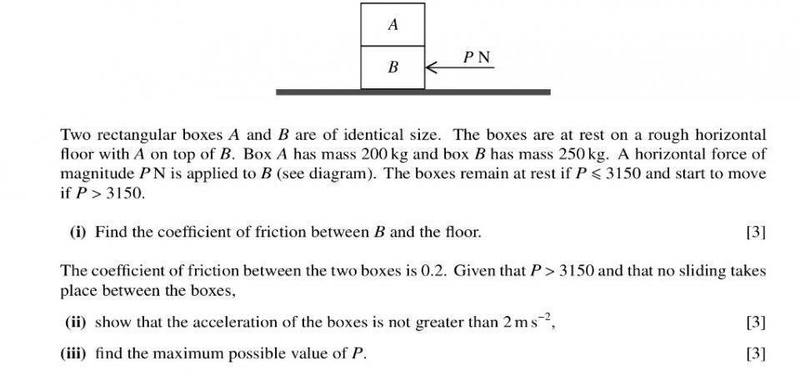# Calculate max acceleration such that A does not slide

Janiceleong26
1. Homework StatementFriction ≤ μR

## The Attempt at a Solution

:[/B]
Ok so I need help for (ii) and this is what I did:
I thought that in order for A not to slide, the net force on B ≤ Friction between the boxes
450a ≤ 0.2(200g)
a≤0.89

however, when I tried this,
200a≤0.2(200g)
a≤2

What's the correct physics behind this?

Homework Helper
Friction ≤ μR might be correct. You don't say what R is ?
What ##\mu## did you find in part (i) (just checking) ?

450 a ≤ 0.2(200g) would that be 200 g like in 200 times the acceleration from gravity ? Again, you don't say what 200 g stands for...

Janiceleong26
Friction ≤ μR might be correct. You don't say what R is ?
What ##\mu## did you find in part (i) (just checking) ?

450 a ≤ 0.2(200g) would that be 200 g like in 200 times the acceleration from gravity ? Again, you don't say what 200 g stands for...
Hi. R is the normal reaction force, sorry for not stating it
I got μ=0.7 for part (i)
200g is the normal reaction force of A due to its weight , a is the acceleration of the the whole body

Homework Helper
So if you draw a free body diagram of A, you get a maximum friction force of mA g Newton. The force to accelerate block A should therefore not be bigger than that. And that force is mA times the acceleration. The mass of B does not come into the equations, only the maximum force it can exert on A.

Janiceleong26
So if you draw a free body diagram of A, you get a maximum friction force of mA g Newton. The force to accelerate block A should therefore not be bigger than that. And that force is mA times the acceleration. The mass of B does not come into the equations, only the maximum force it can exert on A.
Ah I see. So the force that actually accelerates A is the net force, P-(friction between B and floor), right?

Homework Helper
So the force that actually accelerates A is friction force between A and B. that is the only horizontal force that works on A.
the net force, P-(friction between B and floor),
No. If you want to see why not, draw a free body diagram of box B !

Janiceleong26
So the force that actually accelerates A is friction force between A and B. that is the only horizontal force that works on A.
No. If you want to see why not, draw a free body diagram of box B !
But how does frictional force accelerates A? I thought there must be some forward force?

Biker
But how does frictional force accelerates A? I thought there must be some forward force?
The static friction between the boxes will accelerate box ##A##.
There is a forward force acting on ##A##. Imagine this, instead of saying that box ##B## is moving forward. You could reverse this and say that box ##A## is moving backwards. It is just saying that box ##A## is moving backward relative to box ##B##.
And What is the friction direction if an object is trying to move backward? The direction is Forward. The static friction wants box ##A## to keep up with box ##B##.

Another way to imagine this (which I like the most), You could put two fingers on your left hand. Now try to move your left hand in any direction. You will feel a tendency or a force acting on your fingers in the same direction.

Now you may ask why is it static friction not kinetic? Well, you want it to move with the same acceleration, don't you? so as long as the acceleration of box ##A## equals the acceleration of box. We can say that at any moment box ##A## is still relative to box ##B##. You could visualize this two by watching two cars racing each other. The drivers see each other as if they were standing still but they see their surrounding move.

Last edited: